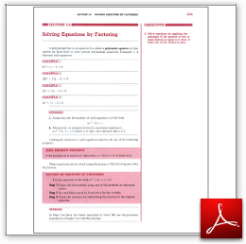Algebra Math Book - Introductory Algebra Thank you for your continual support.

 The power of mathematics rests in the logic of thinking.ID: Sec5-6
Description: Zero Product Property-Solving by Factoring
Price: 1.99

# Algebra Math book - Introductory Algebra - Chapter 5 - Section 6 - Solving Equations by Factoring

## Section 5.6 - Objectives

8.  Solve equations by applying the principle:  If the product of two or more factors is equal to 0, then at least one of the factors is zero.

Navigate to
Previous Section or Next Section
or Chapter 5 Details or Other Chapters

This section of my algebra math book, Introductory Algebra, also includes in the download:

• Cover Sheet